# Generating sets for subgroups of symmetric group:S3

This article gives specific information, namely, generating sets for subgroups, about a particular group, namely: symmetric group:S3.
View generating sets for subgroups of particular groups | View other specific information about symmetric group:S3

This article provides summary information on various choices of generating set for subgroups of symmetric group:S3. It builds on basic information available at element structure of symmetric group:S3 and subgroup structure of symmetric group:S3.

## Probability of generation

The rule is as follows. Given$k$ (not necessarily distinct) elements picked uniformly at random and independently of each other from a finite group$G$, the probability that they all live in a fixed subgroup of index$d$ is$1/d^k$.

Using this and a form of Mobius inversion on the subgroup lattice, it is possible to compute the probability that they generate a fixed subgroup of index$d$ (we basically need to subtract off probabilities for smaller subgroups).

### Generated by one element

Here, a single element is picked uniformly at random from the group.

Automorphism class of subgroups List of all subgroups Isomorphism class Order of subgroups Index of subgroups Probability that an element is in a fixed subgroup of this automorphism class(= reciprocal of index) Probability of generating the subgroup conditional to being in the subgroup (depends only on isomorphism class) Probability that an element generates a fixed subgroup of this automorphism class (product of preceding two columns) Size of automorphism class Probability that the element generates a subgroup in this automorphism class
trivial subgroup$\{ () \}$ trivial group 1 6 1/6 1 1/6 1 1/6
S2 in S3$\{ (), (1,2) \}$$\{ (), (2,3) \}$$\{ (), (1,3) \}$
cyclic group:Z2 2 3 1/3 1/2 1/6 3 1/2
A3 in S3$\{ (), (1,2,3), (1,3,2) \}$ cyclic group:Z3 3 2 1/2 2/3 1/3 1 1/3
whole group$\{ (), (1,2,3), (1,3,2),$$(1,2), (1,3), (2,3) \}$
symmetric group:S3 6 1 1 0 0 1 0
Total (4 rows) -- -- -- -- -- -- -- 6 1

### Generated by two independent possibly equal elements

Here, two elements are picked uniformly at random from the group, independent of each other. They could be equal.

Automorphism class of subgroups List of all subgroups Isomorphism class Order of subgroups Index of subgroups Probability that the elements are in a fixed subgroup of this automorphism class(= reciprocal square of index) Probability of generating the subgroup conditional to being in the subgroup Probability that the elements generates a fixed subgroup of this automorphism class (obtained by multiplying preceding two columns) Size of automorphism class Probability that the elements generates a subgroup in this automorphism class
trivial subgroup$\{ () \}$ trivial group 1 6 1/36 1 1/36 1 1/36
S2 in S3$\{ (), (1,2) \}$$\{ (), (2,3) \}$$\{ (), (1,3) \}$
cyclic group:Z2 2 3 1/9 3/4 1/12 3 1/4
A3 in S3$\{ (), (1,2,3), (1,3,2) \}$ cyclic group:Z3 3 2 1/4 8/9 2/9 1 2/9
whole group$\{ (), (1,2,3), (1,3,2),$$(1,2), (1,3), (2,3) \}$
symmetric group:S3 6 1 1 1/2 1/2 1 1/2
Total (4 rows) -- -- -- -- -- -- -- 6 1

### Generated by$k$ elements picked independently and uniformly at random

Below are given the expressions for general$k$.

Automorphism class of subgroups List of all subgroups Isomorphism class Order of subgroups Index of subgroups Probability that the elements are in a fixed subgroup of this automorphism class(= reciprocal of index raised to$k^{th}$ power) Probability that the elements generates a fixed subgroup of this automorphism class (obtained by Mobius inversion on preceding column) Size of automorphism class Probability that the elements generates a subgroup in this automorphism class
trivial subgroup$\{ () \}$ trivial group 1 6$1/6^k$$1/6^k$ 1$1/6^k$
S2 in S3$\{ (), (1,2) \}$$\{ (), (2,3) \}$$\{ (), (1,3) \}$
cyclic group:Z2 2 3$1/3^k$$1/3^k - 1/6^k$ 3$1/3^{k-1} - 3/6^k$
A3 in S3$\{ (), (1,2,3), (1,3,2) \}$ cyclic group:Z3 3 2$1/2^k$$1/2^k - 1/6^k$ 1$1/2^k - 1/6^k$
whole group$\{ (), (1,2,3), (1,3,2),$$(1,2), (1,3), (2,3) \}$
symmetric group:S3 6 1 1$1 - 1/2^k - 1/3^{k-1} + 3/6^k$ 1$1 - 1/2^k - 1/3^{k-1} + 3/6^k$
Total (4 rows) -- -- -- -- -- -- -- 1

## Small generating sets for subgroups

For symmetric group:S3, the following are equivalent for any subset:

We list below all the small generating sets:

Subset Size Subgroup it generates Is it a generating set of minimum size for the subgroup it generates? (if Yes, size at most 2) Is it a minimal generating set for the subgroup it generates? (if Yes, size at most 2) Is it a Jerrum-reduced generating set? (if Yes, size at most 2) Is it a Sims-reduced generating set? (if Yes, size at most 3)$\{ \}$ 0 trivial subgroup Yes Yes Yes Yes$\{ (1,2) \}$ 1$\{ (), (1,2) \}$ Yes Yes Yes Yes$\{ (1,3) \}$ 1$\{ (), (1,3) \}$ Yes Yes Yes Yes$\{ (2,3) \}$ 1$\{ (), (2,3) \}$ Yes Yes Yes Yes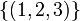$\{ (1,2,3) \}$ 1$\{ (), (1,2,3), (1,3,2)\}$ Yes Yes Yes Yes$\{ (1,3,2) \}$ 1$\{ (), (1,2,3), (1,3,2)\}$ Yes Yes Yes Yes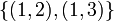$\{ (1,2), (1,3) \}$ 2 whole group Yes Yes No Yes$\{ (1,2),(2,3)\}$ 2 whole group Yes Yes Yes Yes$\{ (1,3), (2,3)\}$ 2 whole group Yes Yes Yes Yes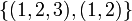$\{ (1,2,3), (1,2) \}$ 2 whole group Yes Yes No No$\{ (1,2,3), (1,3) \}$ 2 whole group Yes Yes No Yes$\{ (1,2,3), (2,3) \}$ 2 whole group Yes Yes Yes Yes$\{ (1,3,2), (1,2) \}$ 2 whole group Yes Yes Yes No$\{ (1,3,2), (1,3) \}$ 2 whole group Yes Yes No No$\{ (1,3,2), (2,3) \}$ 2 whole group Yes Yes Yes Yes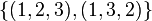$\{ (1,2,3), (1,3,2) \}$ 2 A3 in S3 No No No Yes$\{ (1,2), (1,3), (2,3) \}$ 3 whole group No No No Yes$\{ (1,2,3), (1,3), (2,3) \}$ 3 whole group No No No Yes$\{ (1,2), (1,3,2), (2,3) \}$ 3 whole group No No No Yes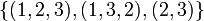$\{ (1,2,3), (1,3,2), (2,3) \}$ 3 whole group No No No Yes

All other subsets fail each of the four questions, so they are not listed.